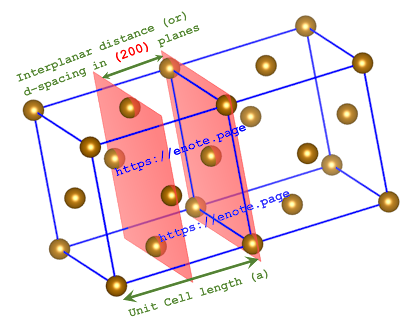## Posts

Showing posts with the label Simulating unit cell dimensions from XRD data

### XRD CUBIC - Simulation of Unit cell dimension, (h, k, l) - Miller indices d-spacing from X-ray diffraction (XRD) angle, 2-theta (For Cubic crystals)Designed a basic computer program “XRD CUBIC” (coded in Python)  to simulate possible unit crystal cell length (a), Miller indices - {h, k, l} and interplanar spacing (d) for cubic crystals from observed (experimental) X-ray diffraction (XRD) angle that is 2-theta.   It can simulate all these possible crystal lattice parameters for cubic crystal systems such as Simple Cubic or Face Centered Cubic or Body Centered Cubic systems, between the given 2–theta values within the range of specified cell length (a) limits.     1. Enter wavelength of X-ray (in Angstroms) 2. Enter minimum and maximum diffraction angle as 2-theta (in Degrees) 3. Enter minimum and maximum unit cell length (in Angstroms) All the possible cubic crystal parameters as well as diffraction angle with reference to d-spacing between the given Miller indices (h k l planes) will be simulated. Video Tutorial   Designed by: Dr. M Kanagasabapathy, Asst. Professor Departm